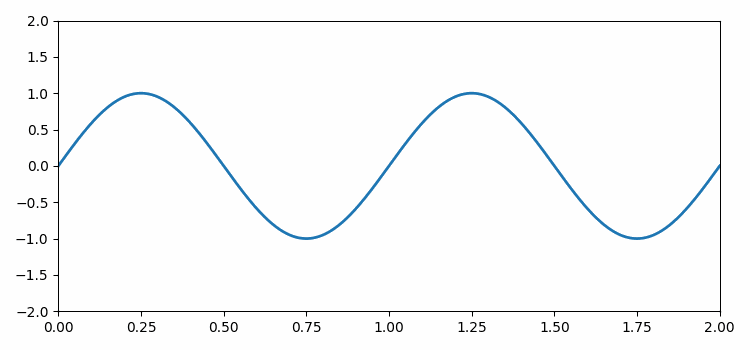# Creating animated GIF files out of D3.js animations in Matplotlib

To create animated GIF files out of D3.js animation, we can take the following steps −

• Set the figure size and adjust the padding between and around the subplots.
• Create a new figure or activate an existing figure.
• Add an axes to the current figure and make it the current axes.
• Plot a line with empty lists.
• To initialize the line, pass empty lists.
• To animate the sine curve, update the sine curve values and return the line instance.
• Get a movie writer instance using PillowWriter() class.
• Save the .gif file using PillowWriter.

## Example

import numpy as np
from matplotlib import pyplot as plt
from matplotlib import animation

plt.rcParams["figure.figsize"] = [7.50, 3.50]
plt.rcParams["figure.autolayout"] = True

fig = plt.figure()
ax = plt.axes(xlim=(0, 2), ylim=(-2, 2))
line, = ax.plot([], [], lw=2)

def init():
line.set_data([], [])
return line,

def animate(i):
x = np.linspace(0, 2, 1000)
y = np.sin(2 * np.pi * (x - 0.01 * i))
line.set_data(x, y)
return line,

ani = animation.FuncAnimation(fig, animate, init_func=init, frames=200, interval=20, blit=True)

writer = animation.PillowWriter(fps=25)

ani.save("sine.gif", writer=writer)

## Output